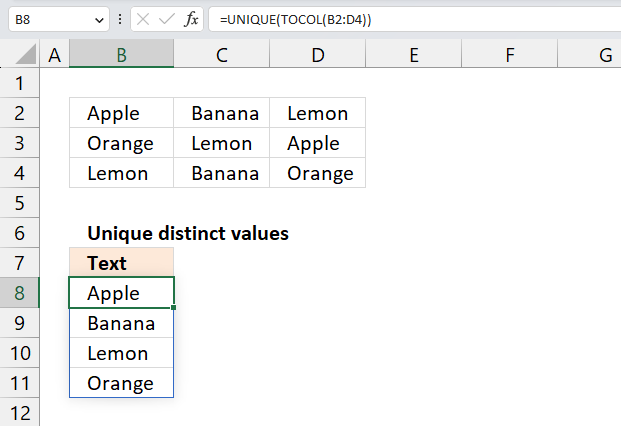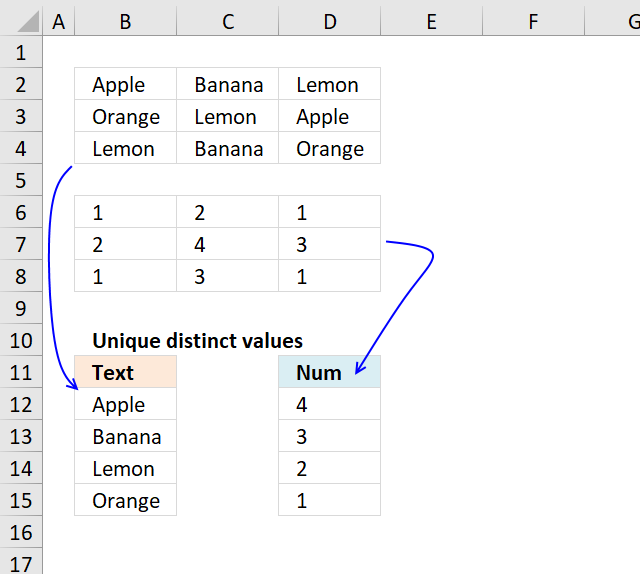Author: Oscar Cronquist Article last updated on May 04, 2023This article demonstrates ways to list unique distinct values in a cell range with multiple columns. The data is not arranged so values belong to each other row by row, this article demonstrates how to list unique distinct rows.

## 1. Extract unique distinct values from a multi-column cell range - Excel 365Excel 365 formula in cell B8:

=UNIQUE(TOCOL(B2:D4))

### Explaining formula in cell B8

#### Step 1 - Rearrange data to a single column array

The TOCOL function rearranges values in 2D cell ranges to a single column.

Function syntax: TOCOL(array, [ignore], [scan_by_col])

TOCOL(B2:D4)

#### Step 2 - List unqiue distinct values

The UNIQUE function returns a unique or unique distinct list.

Function syntax: UNIQUE(array,[by_col],[exactly_once])

UNIQUE(B2:D4)

## 2. Extract unique distinct values from a multi-column cell range - earlier Excel versionsQuestion: I have cell values spanning over several columns and I want to create a unique list from that range. How?

Thanks to Eero, who contributed the original array formula!Unique distinct text values from range tbl_text, array formula in B13:

=INDEX(tbl_text, MIN(IF(COUNTIF(\$B\$12:B12, \$B\$2:\$D\$4)=0, ROW(\$B\$2:\$D\$4)-MIN(ROW(\$B\$2:\$D\$4))+1)), MATCH(0, COUNTIF(\$B\$12:B12, INDEX(\$B\$2:\$D\$4, MIN(IF(COUNTIF(\$B\$12:B12, \$B\$2:\$D\$4)=0, ROW(\$B\$2:\$D\$4)-MIN(ROW(\$B\$2:\$D\$4))+1)), , 1)), 0), 1)

### 2.1 How to enter an array formula

1. Double press with left mouse button on cell B13.
2. Copy and paste above formula to cell B13.
3. Press and hold CTRL + SHIFT simultaneously.
4. Press Enter once.
5. Release all keys.

Your formula now looks like this: {=array_formula}

Don't enter these characters yourself, they appear automatically when you do above steps.

### 2.2 How to copy array formula

Copy cell B13 and paste it down as far as necessary.

### 2.3 Explaining array formula in cell B13

The array formula has two parts. One part returns row numbers and the other part returns column numbers. Let us begin with the first part, returning row numbers.

#### Step 1 - Find new unique distinct text values

The COUNTIF function calculates the number of cells that is equal to a condition.

Function syntax: COUNTIF(range, criteria)

COUNTIF(\$B\$12:B12, tbl_text)=0

becomes

COUNTIF("Text", {"Apple","Banana","Lemon";"Orange","Lemon","Apple";"Lemon","Banana","Orange"})=0

becomes

{0,0,0;0,0,0;0,0,0}=0

and returns

{TRUE,TRUE,TRUE;TRUE,TRUE,TRUE;TRUE,TRUE,TRUE}.

#### Step 2 - Convert boolean array to row numbers

The COUNTIF function calculates the number of cells that is equal to a condition.

Function syntax: COUNTIF(range, criteria)

IF(COUNTIF(\$B\$12:B12, tbl_text)=0, ROW(tbl_text)-MIN(ROW(tbl_text))+1)

becomes

IF({TRUE,TRUE,TRUE;TRUE,TRUE,TRUE;TRUE,TRUE,TRUE}, {2;3;4}-MIN({2;3;4})+1)

becomes

IF({TRUE,TRUE,TRUE;TRUE,TRUE,TRUE;TRUE,TRUE,TRUE}, {2;3;4}-MIN({2;3;4})+1)

becomes

IF({TRUE,TRUE,TRUE;TRUE,TRUE,TRUE;TRUE,TRUE,TRUE}, {2;3;4}-2+1)

becomes

IF({TRUE,TRUE,TRUE;TRUE,TRUE,TRUE;TRUE,TRUE,TRUE}, {1;2;3})

and returns

{1,1,1;2,2,2;3,3,3}

#### Step 3 - Extract smallest value in array

The MIN function returns the smallest number in a cell range.

Function syntax: MIN(number1, [number2], ...)

MIN(IF(COUNTIF(\$B\$12:B12, tbl_text)=0, ROW(tbl_text)-MIN(ROW(tbl_text))+1))

becomes

MIN({1,1,1;2,2,2;3,3,3})

and returns 1.

#### Step 4 - Part two, identify array values in current row

The INDEX function returns a value or reference from a cell range or array, you specify which value based on a row and column number.

Function syntax: INDEX(array, [row_num], [column_num])

INDEX(tbl_text, MIN(IF(COUNTIF(\$B\$12:B12, tbl_text)=0, ROW(tbl_text)-MIN(ROW(tbl_text))+1)), , 1))

becomes

INDEX(tbl_text, MIN({1,1,1;2,2,2;3,3,3}), , 1))

becomes

INDEX(tbl_text, 1, , 1))

and returns array

{"Apple", "Banana", "Lemon"

#### Step 5 - Find new unique distinct text values in current row

The COUNTIF function calculates the number of cells that is equal to a condition.

Function syntax: COUNTIF(range, criteria)

COUNTIF(\$B\$12:B12, INDEX(tbl_text, MIN(IF(COUNTIF(\$B\$12:B12, tbl_text)=0, ROW(tbl_text)-MIN(ROW(tbl_text))+1)), , 1))

becomes

COUNTIF("Text", {"Apple", "Banana", "Lemon"})

and returns

{0,0,0}

#### Step 6 - Find a new unique distinct text value in current row

The MATCH function returns the relative position of an item in an array that matches a specified value in a specific order.

Function syntax: MATCH(lookup_value, lookup_array, [match_type])

MATCH(0, COUNTIF(\$B\$12:B12, INDEX(tbl_text, MIN(IF(COUNTIF(\$B\$12:B12, tbl_text)=0, ROW(tbl_text)-MIN(ROW(tbl_text))+1)), , 1)), 0)

becomes

MATCH(0, {0,0,0}, 0)

and returns 1.

#### Step 7 - Get value

The INDEX function returns a value or reference from a cell range or array, you specify which value based on a row and column number.

Function syntax: INDEX(array, [row_num], [column_num])

INDEX(tbl_text, MIN(IF(COUNTIF(\$B\$12:B12, tbl_text)=0, ROW(tbl_text)-MIN(ROW(tbl_text))+1)), MATCH(0, COUNTIF(\$B\$12:B12, INDEX(tbl_text, MIN(IF(COUNTIF(\$B\$12:B12, tbl_text)=0, ROW(tbl_text)-MIN(ROW(tbl_text))+1)), , 1)), 0), 1)

becomes

INDEX(tbl_text, 1, 1)

and returns value "Apple" in cell B13.### Explaining array formula in cell D13

=LARGE(IF(COUNTIF(\$D\$12:D12, tbl_num)=0, tbl_num, ""), 1)

#### Step 1 - Remove previously extracted values above current cell with an array with boolean values

The COUNTIF function calculates the number of cells that is equal to a condition.

Function syntax: COUNTIF(range, criteria)

COUNTIF(\$D\$12:D12, tbl_num)=0

becomes

{0,0,0;0,0,0;0,0,0}=0

becomes

{TRUE,TRUE,TRUE;TRUE,TRUE,TRUE;TRUE,TRUE,TRUE}

#### Step 2 - Convert boolean values to numeric values

The IF function returns one value if the logical test is TRUE and another value if the logical test is FALSE.

Function syntax: IF(logical_test, [value_if_true], [value_if_false])

IF(COUNTIF(\$D\$12:D12, tbl_num)=0, tbl_num, "")

becomes

IF({TRUE,TRUE,TRUE;TRUE,TRUE,TRUE;TRUE,TRUE,TRUE}, {1, 2, 1;2, 4, 3;1, 3, 1}, "")

and returns

{1, 2, 1;2, 4, 3;1, 3, 1}

#### Step 3 - Convert boolean values to numeric values

The LARGE function calculates the k-th largest value from an array of numbers.

Function syntax: LARGE(array, k)

LARGE(IF(COUNTIF(\$D\$12:D12, tbl_num)=0, tbl_num, ""), 1)

becomes

LARGE({1, 2, 1;2, 4, 3;1, 3, 1}, 1)

and returns 4 in cell D13.

### Get the Excel fileUnique-distinct-values-from-multiple-columns-using-array-formulas1.xls